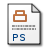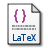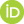# Inertial frames, special relativity and consistency

Srinivasan, Radhakrishnan (2002) Inertial frames, special relativity and consistency. [Preprint]Preview
Postscript
ifr.psTex/LaTeX
ifr.tex

## Abstract

The theory of special relativity (SR) is considered in the framework of classical first-order logic. The four axioms of SR are: The existence of a flat four-dimensional spacetime continuum, the existence of global inertial frames of reference and Einstein's two postulates. The propositions permitted are those involving the kinematics and dynamics of SR as formulated from an inertial frame; these give a complete description of the evolution of the universe. Assuming SR as consistent, there must exist a model M for SR in which F is an inertial frame in a non-trivial universe U(IBC) of material objects, with appropriate initial-boundary conditions IBC specified. Let P be defined as ``F is an inertial frame in U(IBC)''. Using Goedel's second incompleteness theorem, it is argued that P is undecidable in SR. Let Q be any proposition such that (Q --> P) is not a theorem of SR. If, in addition, (P --> Q) is a theorem of SR then Q must necessarily be true in M. It follows that there must exist a model N for SR in which Q is true and P is false, i.e., F is an accelerated frame in U(IBC). The philosophical and mathematical implications of this result for the consistency of SR are discussed.

 Export/Citation: EndNote | BibTeX | Dublin Core | ASCII/Text Citation (Chicago) | HTML Citation | OpenURL
 Social Networking:

Item Type: Preprint
Creators:
CreatorsEmailORCIDCommentary on: Srinivasan, Radhakrishnan (2002) Quantum superposition justified in a new non-Aristotelian finitary logic. [Preprint]
Keywords: inertial frames, consistency, Goedel's incompleteness theorems, self-reference, classical logic
Subjects: Specific Sciences > Mathematics
Specific Sciences > Physics
Specific Sciences > Physics > Relativity Theory
Date Deposited: 27 Jun 2002
Item ID: 666
Subjects: Specific Sciences > Mathematics
Specific Sciences > Physics
Specific Sciences > Physics > Relativity Theory
Date: June 2002
URI: http://philsci-archive.pitt.edu/id/eprint/666View Item